PetroSkills Blog
By:

# The Diffusivity Equation

## Fluid Flow in Porous Media

The theory of well testing begins with an understanding of fluid flow in porous media. In this article, the continuity equation, Darcy’s law, and equation of state for a slightly compressible liquid are used to develop the diffusivity equation, describing single-phase flow of a slightly compressible liquid.

You will learn:

►The three physical principles used to develop the diffusivity equation.

The diffusivity equation describes the flow of a slightly compressible liquid through a porous medium. The diffusivity equation is derived by combining three physical principles:

1) the continuity equation

2) Darcy’s law

3) the equation of state for a slightly compressible liquid

For convenience, we will use a consistent system of units throughout this article.

The Continuity Equation

The continuity equation is a statement of the law of conservation of matter relating the net rate of mass influx into an infinitesimal volume to the rate of accumulation of mass within the volume as illustrated in Figure 2.1. For one-dimensional linear flow, the continuity equation may be written (in consistent units) as: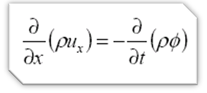Equation 2.1

The velocity ux in the continuity equation is the superficial velocity, defined as the volumetric flow rate per unit cross sectional area, ux = q/A. The average velocity of the fluid is given by vx = u/ Φ.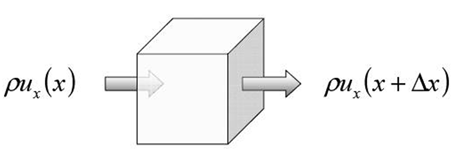Figure 2.1  The continuity equation relates the net rate of mass influx of fluid into an infinitesimal volume to the rate of accumulation of mass within the volume.

Darcy’s Law

Darcy’s law describes the movement of fluid through a porous medium under the influence of a pressure gradient. For constant flow rate q across a core of length L and cross sectional area A, as illustrated in Fig. 2.2, the flow rate and pressure are related by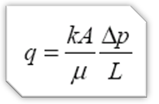Equation 2.2

Where the flow rate q is in cm3/s, the area A is in cm2, the length L is in cm, the pressures p1 and p2 are in atm, the permeability k is in Darcys, and the viscosity μ is in cp. We may write Darcy’s law in differential form as: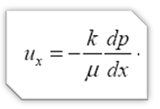Equation 2.3

In differential form, Darcy’s law states that the superficial velocity is proportional to the pressure gradient and opposite in sign, with the constant of proportionality given by the permeability (a property of the porous medium) divided by the viscosity (a property of the fluid). Darcy’s law is an empirical law that holds at low flow velocities, typical of those encountered throughout most of a reservoir. Darcy’s law in differential form, as defined in Eq. 2.3, applies to gases as well as liquids for most reservoir conditions.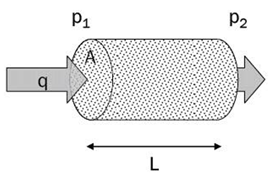Figure 2.2  Linear flow through a core sample.

Equation of State for a Slightly Compressible Liquid

The equation of state for a slightly compressible liquid states that the compressibility, defined as the fractional change in volume per unit change of pressure, is independent of pressure: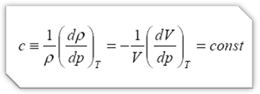Equation 2.4

Eq. 2.4 is a good approximation for the behavior of oil or water under single-phase conditions. It may also be used for gas wells if the drawdown is small, so that all pressures lie within a fairly narrow range such that the gas properties remain approximately constant. Integrating Eq. 2.4 gives the density and volume as functions of pressure: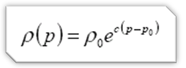Equation 2.5

and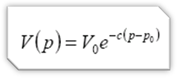Equation 2.6

For a “slightly compressible” liquid, we may write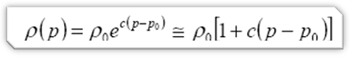Equation 2.7

and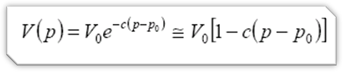Equation 2.8

What exactly is “slightly compressible”? One useful rule of thumb is that the compressibility can be considered “small” if the first neglected term in the Taylor series approximations in Eqs. 2.7 and 2.8 is much less than 1. When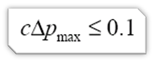Equation 2.9

the first neglected term will be approximately 0.005 or less. For single-phase oil and water wells, Eq. 2.9 will always be satisfied. For a gas well, we can replace the compressiblity in Eq. 2.9 with the ideal gas approximation to obtain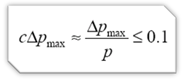Equation 2.10

Thus, if the maximum drawdown during a test is less than 10% of the initial pressure, we may use the liquid equations for a gas well, regardless of the reservoir pressure.

Diffusivity Equation

Combining equations 2.1, 2.3, and 2.4 gives the diffusivity equation for one-dimensional, linear flow of a slightly compressible liquid (in consistent units):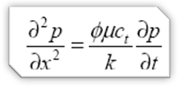Equation 2.11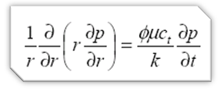Equation 2.12

The diffusivity equation is a linear partial differential equation, first order in time and second order in space. Because the diffusivity equation is linear, we can apply techniques such as the principle of superposition in space and time, Laplace and Fourier transforms, and Green’s functions to obtain solutions to the diffusivity equation for a wide variety of different boundary conditions.

Practicle Example

During a pressure build-up test, we will collect a series of pressures (p) at different times (t) using maybe a pressure guage lowered into the well.  We will assume that the pressures recorded by the tool are very close to the pressures at the wellbore radius(r ) of the well.  This provides us with values for most of the variables in the diffusivity equation except the ones in this expression: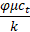If we use values for porosity, viscosity and compressibility drawn from other sources, then we can use the diffusivity equation to calculate the permeability of the reservoir near the well.

This number is extremely useful for predicting the future performance of the well.

To learn more about the diffusivity equation and well test design, we recommend enrolling in the upcoming session of Well Test Design and Analysis - WTA. This course stresses the practical application of well test theory to design and interpret pressure transient tests. View the Reservoir Engineering training schedule for related courses.

## Sidebar

### Featured posts###### Hydrogen Series Part 1: Hydrogen Colors – What do they mean, Options for Production and their associated Challenges?
by Kindra Snow-McGregor

### Popular tags

#Renewables #LNG #Unconventionals